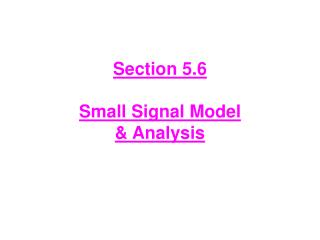DownloadDownload PresentationSection 5.6 Small Signal Model & Analysis

# Section 5.6 Small Signal Model & Analysis

Download Presentation## Section 5.6 Small Signal Model & Analysis

- - - - - - - - - - - - - - - - - - - - - - - - - - - E N D - - - - - - - - - - - - - - - - - - - - - - - - - - -
##### Presentation Transcript

1. Section 5.6Small Signal Model & Analysis

2. Quiz No 1 DE 27 (CE) 06-03-07 State the purpose and four steps (each) that are to be taken for carrying out • DC Analysis (b) Small signal Analysis

3. The operation of the transistor as an amplifier.

4. DC Analysis Signal source eliminated Active Mode Verification VC>VB-0.4 V

5. The collector Current & Trans-conductance

6. The collector Current & Trans-conductance For vbe<< VT, the transistor behaves as a voltage-controlled current device The trans-conductance of the controlled source is gm Output resistance is infinity

7. Base Current & Input Resistance at the Base

8. Emitter Current & Input Resistance @ Emitter

9. Voltage Gain

10. DC-AC Models

11. Large Signal Model Small Signal Model

12. The amplifier circuit

13. Figure 5.51 Two slightly different versions of the simplified hybrid-p model for the small-signal operation of the BJT.

14. Figure 5.52 Two slightly different versions of what is known as the T model of the BJT.

15. Small Signal Analysis • Coupling Capacitors • Couples the input signal vito the emitter while blocks the DC signals • Don’t let dc biasing established by VCC&VEE be disturbed. when vi is connected • Capacitor is of very large value –infinite, acts as short circuit at signal frequency of interest.

16. Application (Steps) : Small Signal Model • Suppress ac independent sources • ac Voltage Sources be short circuited • ac Current Sources be open circuited • Capacitors be Open circuited • Determine DC operating Point IC • Suppress DC independent sources • DC Voltage Sources be short circuited • DC Current Sources be open circuited • Capacitors be short circuited • Replace BJT with small signal Model • Analyze the resulting circuit of find voltage gain & input/output resistance Active Mode Verification VBE > 0.7 V VC> VB-0.4 V Small Signal Analysis

17. The Early Effect • In real world • (a) Collector current does show some dependence on collector voltage • (b) Characteristics are not perfectly horizontal line

18. Figure 5.19 (a) Conceptual circuit for measuring the iC –vCE characteristics of the BJT. (b) The iC –vCE characteristics of a practical BJT.

19. The Early Effect

20. Figure 5.58 The hybrid-pi small-signal model, in its two versions, with the resistance ro included.

21. Problem 5.130 • Find the common-emitter amplifier shown in Fig. P5.130, Let VCC =9V, R1 = 27kΩ, R2 = 15kΩ, RE = 1.2kΩ, and Rc = 2.2kΩ. The transistor has β = 100 and VA = 100 V. Calculate the dc bias current IE. If the amplifier operates between a source for which Rsig = 10 kΩ and a load of 2kΩ replace the transistor with its hybrid-Π model, and find the value of Rm, the voltage gain and the current gain

22. Figure P5.130

23. Figure P5.130 DC Analysis Suppress the AC (independent Sources) Short Circuit Voltage Sources Open Circuit the Capacitors Calculate DC Node Voltages & Loop Currents

24. Figure P5.130 DC Analysis β = 100 , α = 0.99 VA = 100V IE = ?, Rin = ?, overall gain vo/vsig, io/i1

25. Solution P5.130 • DC Values 1.92 mA 9.64 KΩ 3.21 V 1.94 mA

26. 1.92 mA 9.64 KΩ 3.21 V 1.94 mA Solution P5.130 • Check for Mode ACTIVE MODE VCB > - 0.4 V

27. Solution P5.130 • Small Signal Model IC = 1.92 mA VT = 25 mV β = 100 , α = 0.99 VA = 100 V

28. Solution P5.130

29. CE with pi Model

30. CE with ‘T’ Model

31. Comparison ‘pi’ Vs ‘T’ Model

32. Single Stage BJT Amplifier • Three Configurations • Common Emitter (CE) • Common Emitter (CE) with Emitter Resistance • Common Base (CB) • Common Collector (CC)

33. Figure 5.60(a) A common-emitter amplifier using the structure of Fig. 5.59.

34. Amplifiers Configurations Common Emitter

35. Amplifiers Configurations Common Emitter DC Analysis Suppress Independent ac Source Voltage source ----- Short Cct Current Sources --- Open Capacitors ---- Open Cct Redraw the Circuit Analysis VC VB IE=I IC=αIE IB=(β+1)IE VE VC=VCC-ICRC VB=-IBRB VE=VB-VBE gm=Ic/VT rл=β/gm re=α/gm

36. Amplifiers Configurations Common Emitter Small Signal Analysis Suppress Independent DC Source Voltage source ----- Short Cct Current Sources --- Open Capacitors ---- Short Cct Redraw the Circuit by replacing BJT With pi Model Analysis gm=Ic/VT rл=β/gm re=α/gm Find Rin, Rout, Voltage Gain vo/vi

37. Common Emitter + vbe - Short Circuit Current Gain Ais Ais = ios/ii ios=-gmvbe vbe=vi=iiRin Ais=-gmRin Rin=RB||rл Rout=RC|RL

38. Summary : CE Input Resistance Low to moderate typically a few kilohms Output Resistance Output Resistance is relatively low Open Circuit Voltage Gain Voltage gain of a few hundred Short Circuit Current Gain Current gain equal to β

39. Redraw the circuit for DC analysis Redraw the circuit for Small Signal pi model analysis Quiz No 2 (DE 27 CE) 13-03-2007

40. Small Signal Analysis : CE with Emitter ResistanceInput Resistance Multiplication by a factor (1+β) is known as the Resistance Reflection Rule. Analysis

41. Small Signal Analysis : CE with Emitter ResistanceVoltage Gain Voltage gain is lower than that of CE because of the additional term (1+β)Re